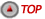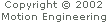### About the PID PIDOutputOffset Parameter

The PIDoutput Offset parameter is a constant value added to the PID calculation before sending the DAC value to the DAC.Reason More 1 To compensate for the input offset errors in a "conventional" amplifier For situations when the amplifier performs the commutation. Individual DAC offsets can also be used to zero out-of-spec amplifier offsets. 2 If a static DC torque (force) on the system is needed Typically used with a gravity-loaded (vertical) axis that has a simple current amplifier controlling the motor 3 For testing the DAC If the axis is aborted or all PID gains are zeroed, you can use the PIDOutputOffset parameter to set the DAC voltage directly. (3277 = 1Volt, 6553 = 2Volts, etc.) You can only use the PIDOutputOffset parameter if the controller is not configured for motor commutation. If the controller is configured for commutation, use the Commutation DAC Offset to test the DAC. 4 For using the DAC as a general purpose analog output In some cases, the easiest way for the host to generate an analog signal is to set all PID gains to zero and use the PIDOutputOffset parameter to set the desired voltage level. Alternatively, you can use the Commutation DAC Offset for the same purpose, which has the advantage that the voltage levels can be individually set for the two DACs.